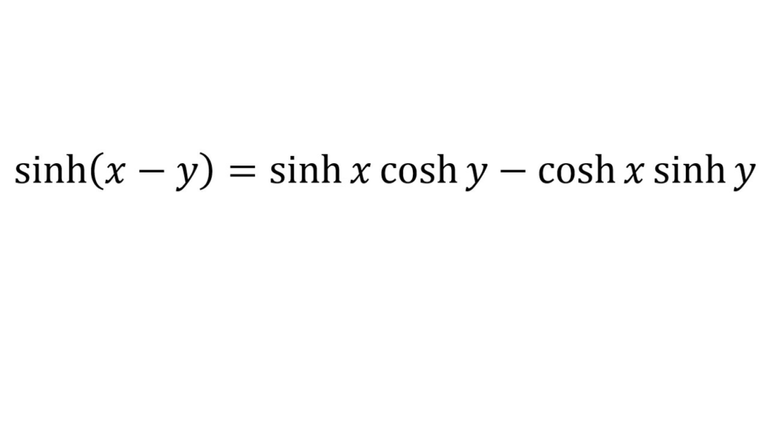# Hyperbolic Trigonometric Identity: sinh(x-y)

in Threespeak2 months ago (edited)In this video I go over a quick derivation of the hyperbolic trig identity sinh(x – y) = sinh(x)cosh(y) – cosh(x)sinh(y). The derivation simply uses my past work on the proof for sinh(x + y) = sinh(x)cosh(y)+cosh(x)sinh(y) and simply replaces y with -y. Thus using the definitions of sinh and cosh I show that cosh(-y) = cosh(y) and sinh(-y) = - sinh(y). Thus the only that changes when compared to the sinh(x+y) identity is we subtract instead of add the two terms together. This is a simple and quick derivation but shows the importance of building up previous work, so make sure to watch this video!

Download the notes in my video: https://1drv.ms/b/s!As32ynv0LoaIhvwoBncxK428IJFN0Q

View video notes on the Hive blockchain: https://peakd.com/mathematics/@mes/3xyhuv-video-notes-hyperbolic-trigonometric-identity-sinh-x-y

Related Videos:

Hyperbolic Trigonometric Identity: sinh(x+y): https://youtu.be/CtBzwqd4Rqc
Hyperbolic Trigonometric Identity: cosh(x-y): https://youtu.be/GMGaQTym4d4
Hyperbolic Trigonometric Identity: cosh(x+y): https://youtu.be/B_rfrnhx-t4
Hyperbolic Functions - tanh(x), sinh(x), cosh(x) - Introduction: http://youtu.be/EmJKuQBEdlc
Hyperbolic Trigonometry Identity Proof: sinh(-x) = -sinh(x), cosh(-x) = cosh(x): http://youtu.be/-Wqq4Wzi7O8 .

SUBSCRIBE via EMAIL: https://mes.fm/subscribe

DONATE! ʕ •ᴥ•ʔ https://mes.fm/donate

Like, Subscribe, Favorite, and Comment Below!

Follow us on:

MES Truth: https://mes.fm/truth
Official Website: https://MES.fm
Hive: https://peakd.com/@mes
Gab: https://gab.ai/matheasysolutions
Minds: https://minds.com/matheasysolutions
Twitter: https://twitter.com/MathEasySolns
Facebook: https://fb.com/MathEasySolutions
LinkedIn: https://mes.fm/linkedin
Pinterest: https://pinterest.com/MathEasySolns
Instagram: https://instagram.com/MathEasySolutions
Email me: [email protected]

Free Calculators: https://mes.fm/calculators

BMI Calculator: https://bmicalculator.mes.fm
Grade Calculator: https://gradecalculator.mes.fm
Mortgage Calculator: https://mortgagecalculator.mes.fm
Percentage Calculator: https://percentagecalculator.mes.fm

Free Online Tools: https://mes.fm/tools

iPhone and Android Apps: https://mes.fm/mobile-apps

▶️ 3Speak